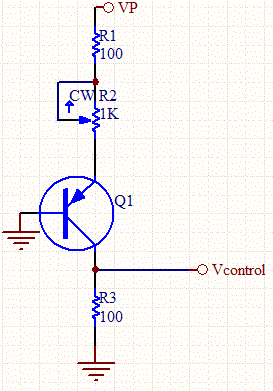# Inverse Voltage Control

This circuit creates a voltage inversely proportional to a variable resistance.The PNP transistor is turned on to saturation. So the voltage through the collector is:

IR1= (VP-VBE)/(R1+R2)

VControl= R3(VP-VBE)/(R1+R2)